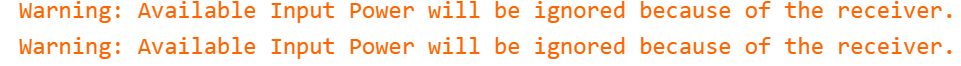# rfantenna

Create antenna element modeled as transmitter and receiver

## Description

Use the `rfantenna` object to create an antenna element that you can model as a transmitter or receiver. You can also use the `rfantenna` object to compute the effective isotropically radiated power (EIRP) in cascaded stages using the RF Budget Analyzer app.

## Creation

### Syntax

``ant = rfantenna``
``ant = rfantenna(Name=Value)``

### Description

````ant = rfantenna` creates a default RF antenna object with a gain of `1` dBi.```

example

````ant = rfantenna(Name=Value)` sets the Properties of an `rfantenna` object using one or more name-value arguments. For example, `ant = attenuator(Gain=10)` creates an RF antenna object with a `10` dBi gain. Properties you do not specify retain their default values.```

## Properties

expand all

Name of the RF antenna element, specified as a string scalar or a character vector.

Example: `rfantenna(Name='Antenna_20dB')`

Antenna gain, specified as a positive scalar in dBi. EIRP can be controlled by varying the gain of the antenna element.

Example: `rfantenna(Gain=20)`

Input impedance of the RF antenna element, specified as a positive scalar in ohms.

Example: `rfantenna(Z=20)`

Type of the antenna element, specified as `Transmitter` or `Receiver`.

Example: `rfantenna(Type='Receiver')`

EIRP value of the transmitting antenna that the receiver is tuned to, specified as a numerical scalar in dBm. You must set this property when you are designing a receiver antenna element.

Example: `rfantenna(TxEIRP=24)`

Loss encountered by a signal before reaching the receiver, specified as a positive scalar in dB.

Example: `rfantenna(PathLoss=2)`

Number of input and output ports in RF antenna element, specified as a positive scalar.

Terminals of RF antenna, specified as a cell array.

## Object Functions

 `sparameters` Calculate S-parameters for RF data, network, circuit, and matching network objects

## Examples

collapse all

Create an amplifier with the gain of `4` dB.

`a = amplifier(Gain=4);`

Create a modulator with OIP3 of `13` dBm.

`m = modulator(OIP3=13);`

Create an n-port element using `passive.s2p`.

`n = nport('passive.s2p');`

Create an RF antenna with the gain of `10` dB.

`ant = rfantenna(Gain=10);`

Calculate the RF budget of a series of RF elements at the input frequency of` 2.1` GHz, available input power of `–30` dBm, and bandwidth of `10` MHz. EIRP is computed under `Analyzed Results` of the `rfbudget` object.

`b = rfbudget([a m n ant],2.1e9,-30,10e6)`
```b = rfbudget with properties: Elements: [1x4 rf.internal.rfbudget.Element] InputFrequency: 2.1 GHz AvailableInputPower: -30 dBm SignalBandwidth: 10 MHz Solver: Friis AutoUpdate: true Analysis Results OutputFrequency: (GHz) [ 2.1 3.1 3.1 3.1] OutputPower: (dBm) [ -26 -26 -30.6 -30.6] TransducerGain: (dB) [ 4 4 -0.5995 -0.5995] NF: (dB) [ 0 0 1.224 1.224] IIP2: (dBm) [] OIP2: (dBm) [] IIP3: (dBm) [ Inf 9 9 9] OIP3: (dBm) [ Inf 13 8.4 8.4] SNR: (dB) [73.98 73.98 72.75 72.75] EIRP: (dBm) [-20.6] Directivity: (dBi) [ 10] ```

Design an RF receiver antenna element given a transmitting antenna with an EIRP value of –35 dBm and a pathloss of 2 dB.

`antR = rfantenna(Type ='Receiver',TxEIRP =-35,PathLoss=2);`

Create an amplifier with a gain of `4` dB.

`a = amplifier(Gain=4);`

Create a modulator with an OIP3 value of `13` dBm.

`m = modulator(OIP3=13);`

Create an n-port element using `passive.s2p`.

`n = nport('passive.s2p');`

Calculate the RF budget of a series of RF elements by tying this command at the command line with an input frequency of 2.1 GHz, an available input power of –30 dBm, and a bandwidth of 10 MHz.

```b = rfbudget([antR a m n],2.1e9,-30,10e6); ```Use the `show` command at the command line to visualize the RF budget chain in the RF Budget Analyzer app. To do further analysis on this chain using this app see RF Budget Analyzer.

```show(b) ```